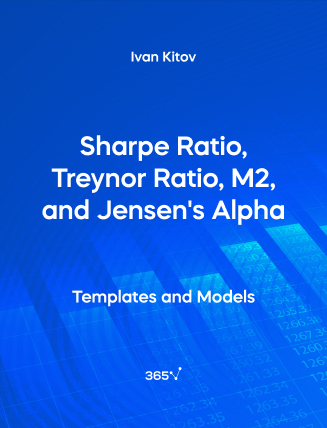## Sharpe Ratio vs Treynor Ratio vs M2 vs Jensen’s Alpha – Excel Template###### Ivan Kitov

Rational investors aim to maximize the rate of return and minimize the risk of investment, so they require a measure of risk-adjusted return to help them compare different securities. Some of the most popular investment performance metrics are—the Sharpe ratio, M squared ratio, Treynor ratio, and Jensen’s alpha.

The Sharpe ratio measures the expected return per unit of risk. It is equal to the excess return of a stock divided by the standard deviation of the stock.

The Treynor Ratio is a portfolio performance measure that illustrates the excess return per unit of systematic risk. It is an extension of the Sharpe ratio but instead of using total risk, it incorporates beta, or systematic risk, in the denominator. In the numerator, you can find the risk premium.

M squared (M²) is a risk-adjusted performance measure that evaluates the performance of an investment portfolio. The idea behind this ratio is to create a synthetic portfolio that mimics the risk of the market portfolio. You can do that by altering the weights of the actual product mix and the risk-free asset until the synthetic portfolio has the same total risk as the market portfolio. Since mimicking a portfolio carries the same risks as the market portfolio, you can use its return as a direct point of comparison to the market return.

Jensen’s alpha finds the difference between the portfolio’s actual return and the required return by the Capital Asset Pricing Model (CAPM). This ratio represents the abnormal return of a security or portfolio over the theoretical expected return predicted by the CAPM. A positive Jensen’s alpha indicates that the portfolio has outperformed the market. On the contrary, a negative result shows that a portfolio has underperformed the market.

This open-access Excel template is a useful tool for statisticians, financial analysts, data analysts, portfolio managers, and anyone working with spreadsheet software.

Sharpe Ratio, Treynor Ratio, M2, and Jensen’s Alpha Treynor Ratio are among the topics included in the Portfolio Management module of the CFA Level 1 Curriculum. Gain valuable insights into the subject with our Portfolio Management course.

You can also explore other related templates such as—Sortino Ratio, Information Ratio, and Variance and Standard Deviation.Top